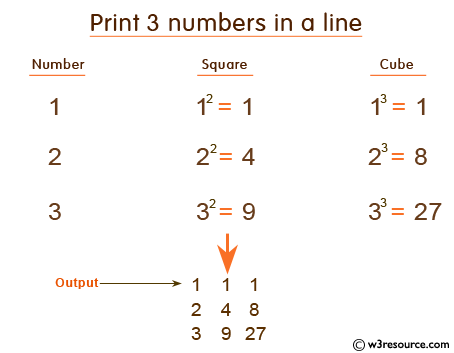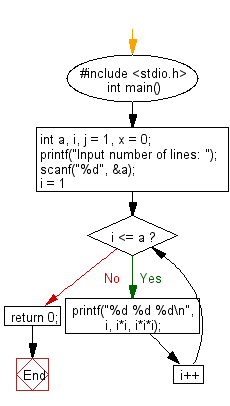﻿ C : Print square and cube in a line from 1 and print n lines

# C Exercises: Print a number, it’s square and cube in a line, starting from 1 and print n lines

## C Basic Declarations and Expressions: Exercise-42 with Solution

Write a C program to print a number, its square and cube, starting with 1 and printing n lines. Accept the number of lines (n, integer) from the user.

Pictorial Presentation:Sample Solution:

C Code:

``````#include <stdio.h>
int main() {
int a, i, j = 1, x = 0;

// Prompt for user input
printf("Input number of lines: ");
scanf("%d", &a);

// Loop for each line
for(i = 1; i <= a; i++) {
// Print i, i squared, and i cubed
printf("%d %d %d\n", i, i*i, i*i*i);
}

return 0;
}
``````

Sample Output:

```Input number of lines: 5
1 1 1
2 4 8
3 9 27
4 16 64
5 25 125
```

Flowchart:C programming Code Editor:

What is the difficulty level of this exercise?

Test your Programming skills with w3resource's quiz.

﻿